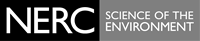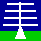THE NERC MST RADAR FACILITY AT ABERYSTWYTH

# Format of MST radar Doppler spectra files

However, users are encouraged to make use of Version-2 MST radar data products.

File contents
The NERC MST radar at Aberystwyth operates in the Doppler Beam Swinging mode, which involves making observations in a cyclic sequence of vertical and near-vertical beam pointing directions (each one known as a dwell). Each file therefore contains range-gated Doppler power spectra for a number of dwells. Where several cycles of observation are stored in a single file (which is typical), the dwell sequence is the same for each cycle.

File naming convention:
dsYYMMDD_hhmm.ddYYis a 2-digit year [00 - 99] MM is a 2-digit month [01 - 12] DD is a 2-digit day [01 - 31] hh is a 2-digit hour [00 - 23] DD is a 2-digit minute [00 - 59] dd is the integer number of minutes covered by the file

Archiving convention: YYYY/mon

File availability
Doppler spectra data are available from 1990 onwards.

File format
The data are stored in non-standard format binary files, as described below. The following is the original file description. Click here to see a more verbose description. Note that some terms and abbreviations differ between the two descriptions.

```
DOPPLER SPECTRA FILES
---------------------

File name :-  DSyyMMdd_hhmm.nn  where yyMMdd is the date
hhmm is start time (UT) and
nn is the duration of the sample in minutes

RANDOM ACCESS FILE  -  UNFORMATTED
RECORD = 64 BYTES

DATA IN FILE
------------

Each DS file contains Doppler Spectra from the MST Radar for every
dwell and cycle in the duration of the sample.

These are quite large data volumes!

When data is aquired on the MST Radar system a setup file
is used to store the data collection parameters.
The radar system uses the information in this setup file to set
the radar parameters for each beam before it starts to collect
data on that beam.
This information is copied to the parameter block for that beam.

Data is collected on a given beam direction for long enough
to collect all the data points required for the FFT length and
FFT bandwidth specified in the setup file.

Up to six beams may be used when collecting data and the data
for each beam is called a Dwell.
Data collected for a set of beams is called a Cycle and usually
there will be more than one Cycle in an Observation.
Therefore each beam collected is labelled with its Dwell and
Cycle number.

The first height range refers to data collected below 30 Km
and the second height range is for data collected above 58 Km.
Height of data collected is referenced by the number of the range bin.
Range bins are at intervals of 150 metres.
To obtain the true height of a given bin an offset must be
subtracted to correct for various time delays in the radar.

RECORD ORDERING
---------------

RECORD 1 :- PARAMETER BLOCK           ( PB )
RECORD 2 :- AUXILIARY PARAMETER BLOCK ( APB )( 1 ONLY )
RECORD 3 :- START OF DOPPLER SPECTRA  ( DSP )

DOPPLER SPECTRA REQUIRE (FFT LENGTH / 64 ) RECORDS.

File layout.
------------

<<<>>>       <<<>>>
PB,APB,DSP,DSP,.....DSP,,PB,FLR,DSP,DSP,.....DSP,etc...

<<<>>>
....DSP,,PB,FLR,DSP,DSP,.....DSP,,TRL

RECORD FORMATS
--------------

Parameter Block ( PB )
----------------------
One record at the start of each Dwell
Uses 44 Bytes out of 64 available.
B = BYTE , I2 = INTEGER*2

Length of Transmitter pulse ( microsec ).                LTX     B
Pulse Code Resolution.                                   NCC     B
0 = Uncoded , 1 = 8 uS , 2 = 4 uS
3 = 2 uS , 4 = 1 uS
Pulse Repetion Interval ( microsec ).                    IPI     I2
Number of Coherent Pulse Additions.                      NPP     I2
Length of FFT.                                           LFT     I2
Number of FFTs Averaged together.                        NAV     I2
Start of First Height Range ( Bin No. ).                 NH1     I2
End of First Height Range ( Bin No. ).                   NH2     I2
Beam Number.                                             NBM     I2
YEAR in Form 90,91,92 etc.                               IY      I2
MONTH ( 1 - 12 )                                         IMN     I2
DAY ( 1 - 31 )                                           ID      I2
HOUR ( 0 - 23 )      ]                                   IH      I2
MINUTE ( 0 - 59 )    ] - Dwell Start time                IM      I2
SECONDS ( 0 - 59 )   ]                                   IS      I2
Start of Second Height Range ( Bin No. ).                NH3     I2
End of Second Height Range ( Bin No. ).                  NH4     I2
Height Interval in Bins (units of 150 metres).           NHI     I2
Receiver Filter (microsec) (1/Bandwidth)                 NRX     B
Raw Data Collection Flag.                                DMP     B
Negative value if Raw Data File Collected.
Dwell Number.                                            NDW     I2
Cycle Number.                                            NCY     I2
Run Number.                                              MST     I2
Number right shifts incorporated in input data.          NRS     I2

Auxiliary Parameter Block ( APB )
---------------------------------

One record only, in the first dwell of each file.
Uses 26 Bytes out of 64
I2 = INTEGER*2 , I4 = INTEGER*4

Number of Dwells per Cycle.                              NDY     I2
No. of Records in Dwell 1.                               NRF(1)  I2
Sum of Records in Dwells 1 and 2.                        NRF(2)  I2
:
:
Sum of Records in  Dwells 1 to 10 inclusive.             NRF(10) I2
Number of Last Record in File.                           NREND   I4

Doppler Spectra ( DSP )
-----------------------

Doppler spectra may be 64, 128, 256 or 512 points (frequencies) long.
They therefore require 1,  2,   4 or   8 records per spectrum (LFT/64).
The total number of records required per Dwell is
where necessary at the end of the dwell.

The spectra start at the most negative Doppler frequency.

At each frequency the power value is given to the nearest 0.2 dB.
Each power value takes up 1 Byte.
A value of 127 represents 0.0 dB.
A value of 0 represents -25.4 dB.
A value of -128 represents -51.0 dB.

To remove ground clutter echoes etc, which can give unwanted signals
at zero Doppler shift (the DC line), the power value at the DC line
is rejected.

The spectra are normalised so that the maximum value in each spectrum
is 0.0 dB. The Scaling Factor ( SF ) used in this normalisation is
written within the spectrum by replacing the rejected power value at
the DC line with SF.

To restore a clean and un-normalised spectrum the SF value must be used
and then replaced by the average of the two power values from
each side of the DC line.

The DC line is the centre frequency of the spectrum at ( LFT / 2 ) + 1.

The Scaling factor is in units of 0.5 dB with a -32.0 dB offset.
( A value of -64 units therefore is equal to 0.0 dB ).

Power at each frequency is therefore :-
((Value - 127) * 0.2) + ((SF + 64) * 0.5) ( dB's )

FFT frequency span is :-  1E6 / (IPI * NPP)  ( Hz ).

Filler Record ( FLR )
---------------------

Blank record inserted after every parameter block and also at the end
of a dwell, when necessary to make the number of records even.

Trailer Record ( TRL )
------------------------

End of file flag (0)                                     EOFF    I2
Continuation flag ( 1 or 0 )                             CTFF    I2

HEIGHT DERIVATION
-----------------

Height at range bin n is given by :-  Ht = (n - Bz) * HI

Bz is the sea level range bin,
derived as follows from dwell header parameters:
LTX = Transmitter pulse width (microsecs).
If NRX = 1  Bz = 5.7 ( unless LTX = 1 when Bz = 5.2 )
If NRX = 2  Bz = 6.7
If NRX = 4  Bz = 8.7
If NRX = 8  Bz = 12.7

HI is the height increment per bin.
derived as follows from dwell header parameter:
NBM = Antenna beam number.
If NBM = 0               HI = 0.15 Km.    ( Vertical )
If NBM = 1,3,5 or 7      HI = 0.1496 Km.  ( 4.2 Deg. )
If NBM = 2,4,6 or 8      HI = 0.1484 Km.  ( 8.5 Deg. )
If NBM = 9,11,13 or 15   HI = 0.1492 Km.  ( 6.0 Deg. )
If NBM = 10,12,14 or 16  HI = 0.1467 Km.  ( 12.0 Deg.)

This description last updated 16 February 1995.
```